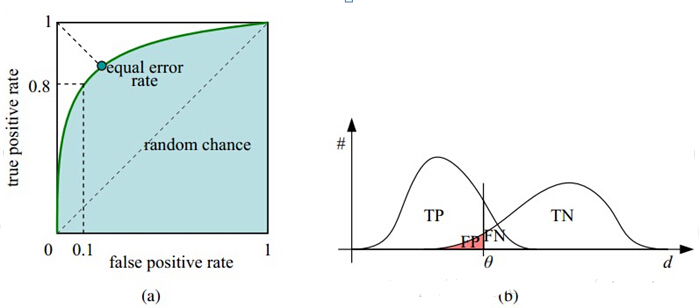# python机器学习案例系列教程——模型评估总结

python数据挖掘系列教程

## 回归评估指标

MSE （Mean Squared Error）叫做均方误差。看公式

$\frac{1}{m}\sum _{i=1}^{m}\left({y}_{i}-f\left({x}_{i}\right){\right)}^{2}$

RMSE（Root Mean Squard Error）均方根误差。

$\sqrt{\frac{1}{m}\sum _{i=1}^{m}\left({y}_{i}-f\left({x}_{i}\right){\right)}^{2}}$

MAE

MAE(平均绝对误差)

$\frac{1}{m}\sum _{i=1}^{m}|{y}_{i}-f\left({x}_{i}\right)|$

## 分类评估

1.1、 混淆矩阵

FN：False Negative,被错误判定为负样本，但事实上是正样本。
​FP：False Positive,被错误判定为正样本，但事实上是负样本。
​TN：True Negative,被正确判定为负样本，事实上也是负样本。
​TP：True Positive,被正确判定为正样本，事实上也是正样本。​

1.2、 准确率及误差率的度量

F、${F}_{1}$$F_1$、F分数精度和召回率的调和均值 $\frac{2\ast 精度\ast 召回率}{精度+召回率}$$\frac{2*精度*召回率}{精度+召回率}$
${F}_{\beta }$$F_β$ $\frac{\left(1+{\beta }^{2}\right)\ast 精度\ast 召回率}{{\beta }^{2}\ast 精度+召回率}$$\frac{(1+β^2)*精度*召回率}{β^2∗精度+召回率}$ β=1退化为${F}_{1}$$F_1$；β>1召回率有更大影响；β<1精度有更大影响。

1.3、 评价模型成本的可视化工具

1.3.1、 lift图

lift叫提升指数，也就是运用模型比不运用模型精度的提升倍数。

$lift=\frac{TP}{TP+FP}/\frac{TP+FN}{P+N}$$lift=\frac{TP}{TP+FP} /\frac{TP+FN}{P+N}$

$lift=\frac{累计预测精度}{平均精度}$$lift=\frac{累计预测精度}{平均精度}$

1.3.2、ROC曲线

ROC曲线是利用真正率（正元组中被准确预测的比例）为纵轴，假正率（预测结果为负元组的样本中实际为正元组的比例）为横轴画出的曲线，用来评估模型预测准确率。很明显，当真正率大越近1，假正率越接近0是模型越好。1.3.3、AUC

AUC(area under curve):即ROC曲线下的面积。

$auc=\frac{所有正面组合数}{m\ast n}$

$AUC=\frac{\sum _{正样本}ran{k}_{i}-\frac{M\left(M+1\right)}{2}}{M\ast N}$

## 1.4、 评估分类器的准确率

1.4.1、 再替换方法

1.4.2、 保持方法和随机子抽样

1.4.3、 交叉验证法

K折交叉验证(K-fold cross-validation):将样本分成K份，每份数量大致相等，然后用其中的某一份作为测试，其他样本作为训练集，得到一个模型和一组预测值及模型评估值；循环这个过程K次，得到K组模型评估值，对其取平均值即得到最终的评估结果；

1.4.4、 自助法

2.1、 统计模型之间的距离

0-1距离

2.2、 统计模型的离差

## 5、计算标准

5.1、 交叉检验（CV）标准

1、Hold-Out Method

2、Double Cross Validation（2-fold Cross Validation，记为2-CV）

3、K-fold Cross Validation（K-折交叉验证，记为K-CV，也叫S折交叉验证）

4、Leave-One-Out Cross Validation（记为LOO-CV，也叫做留一交叉验证）

（1）每一回合中几乎所有的样本皆用于训练模型，因此最接近原始样本的分布，这样评估所得的结果比较可靠。
（2）实验过程中没有随机因素会影响实验数据，确保实验过程是可以被复制的。

5.2、 自展标准
5.3、 遗传算法

## 7、回归模型评估指标

RMSE（root mean square error，平方根误差）：其又被称为RMSD（root mean square deviation），RMSE对异常点（outliers）较敏感，如果回归器对某个点的回归值很不理性，那么它的误差则较大，从而会对RMSE的值有较大影响，即平均值是非鲁棒的。其定义如下：
Quantiles of Errors： 为了改进RMSE的缺点，提高评价指标的鲁棒性，使用误差的分位数来代替，如中位数来代替平均数。假设100个数，最大的数再怎么改变，中位数也不会变，因此其对异常点具有鲁棒性。

## 聚类模型评估

1.1 Adjusted Rand index 调整兰德系数1.2 Mutual Information based scores 互信息1.3 Homogeneity, completeness and V-measure

1.4 Fowlkes-Mallows scores1.5 Silhouette Coefficient 轮廓系数1.6 Calinski-Harabaz Index在scikit-learn中， Calinski-Harabasz Index对应的方法是metrics.calinski_harabaz_score.